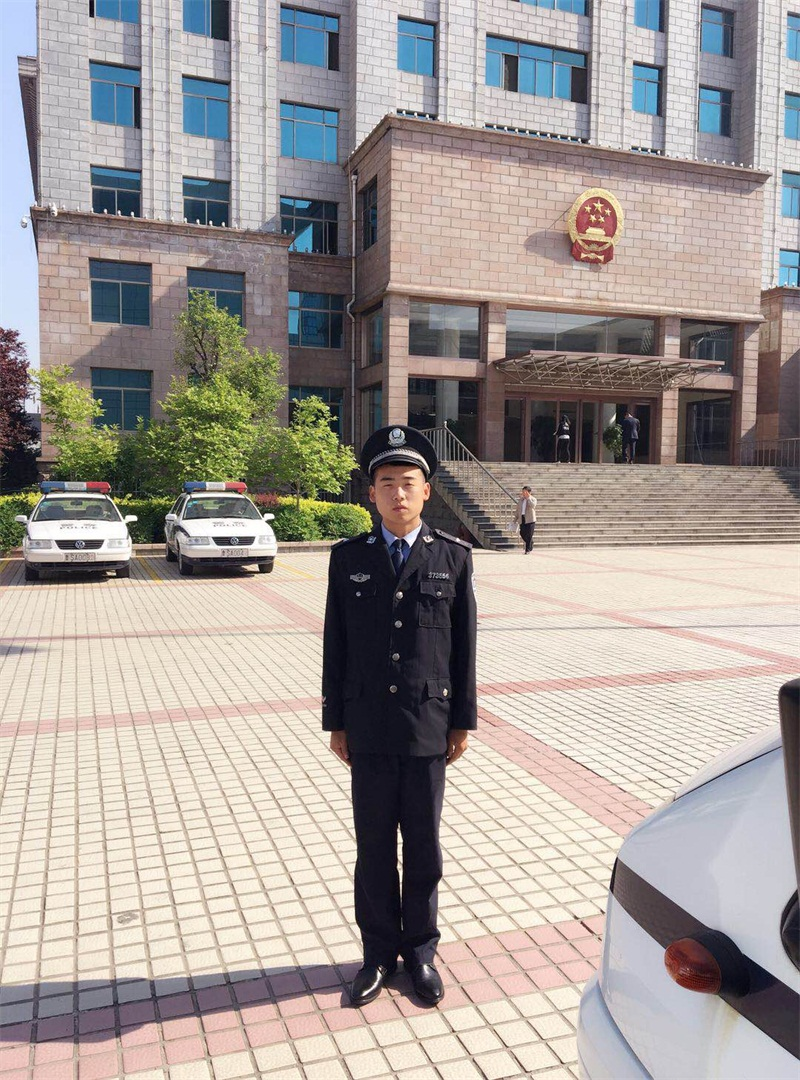# 植树问题之基础理论!

一、问题描述

1、 Problem description

种植的问题其实很简单,是植树的研究在路上,当然,大多数学生能够想到这里,但是在公共考试,这个看似简单的问题如何检查问题,有什么需要注意的地方,种树的问题如何处理,这是我们需要关心的问题。根据试题，植树问题主要研究树木数量与道路距离的关系。

The problem of planting is actually very simple. The research of planting trees is on the way. Of course, most students can think of it here, but in the public examination, how to check the seemingly simple problem, what needs to be paid attention to, and how to deal with the problem of planting trees are the issues we need to be concerned about. According to the test questions, the problem of tree planting mainly studies the relationship between the number of trees and the distance of the road.

第二，问题类型的分类

Second, classification of problem types

在植树造林的问题上，主要有两种情况，一种是在直线上植树，另一种是在封闭的环形线上植树。首先，让我们看看种类型:

In terms of afforestation, there are two main situations, one is to plant trees in a straight line, the other is to plant trees in a closed ring line. First, let's look at the first type:

1. 线性植树问题

1. Linear tree planting

如果你在一条直线上种树，我们来看看树的数量和路的距离之间的关系。举个例子。

If you plant trees in a straight line, let's look at the relationship between the number of trees and the distance of the road. for instance.

例如:在一条100米长的路上，你需要种植多少棵树，只在一边种植，每五米种植一棵，同时在路的两端种植?

For example: on a 100 meter long road, how many trees do you need to plant, only on one side, every five meters, and at both ends of the road?

所以这是一个问题，很多人可能只是凭直觉，把100除以5，但这是一个错误。这个问题,共100米,太多的想象认为,我们来自较少,如两棵树,三棵树的距离会有两个距离,四棵树有三个距离,等等,我们会发现,树的数量总是超过的数量,在这种情况下,我们有这个样本,共100米,5米每一种一棵树,用100除以5等于20,计算的距离是20,树木的数量超过一个的距离,即21树。

So this is a problem, many people may just intuitively divide 100 by 5, but it's a mistake. This problem, 100 meters in total, is too much to imagine that we come from less, such as two trees, three trees will have two distances, four trees have three distances, and so on, we will find that the number of trees is always more than the number, in this case, we have this sample, 100 meters in total, 5 meters each tree, 100 divided by 5 equals 20, and the calculated distance is 20, The number of trees exceeds a distance of 21.结论:在直路上种植的树木数量比距离多1棵。

Conclusion: the number of trees planted on the straight road is 1 more than the distance.

2. 环行植树问题

2. Tree planting around

如果你在一条闭合的环路上种植一棵树和在一条直线上种植一棵树是一样的，或者如果有什么不同我们应该注意到，让我们看看。

If you plant a tree on a closed loop, it's the same as planting a tree on a straight line, or if there's any difference we should notice, let's see.

例如:在一条100米长的环形公路上，每隔5米种一棵树需要多少棵树?

For example: on a 100 meter long ring road, how many trees does it take to plant a tree every 5 meters?

问题是与之前同样的问题,的区别是直线的路到一个密封圈路和我们将分析树的数量和距离,两棵树之间的关系有两个距离,三棵树有三个距离,四棵树有四个距离,同样的我们将得到另一个结论,在环城公路关闭,树木的数量和距离的数量是平等的,这个问题是用100除以5等于20,20棵距离，距离的个数和树的个数相同，即20棵树。

The problem is the same as before. The only difference is that there are two distances between the straight road and a sealed ring road and the number and distance of trees we will analyze. There are two distances between two trees, three trees have three distances, and four trees have four distances. Similarly, we will get another conclusion. When the ring road is closed, the number of trees and the number of distances are equal, The problem is that dividing 100 by 5 is equal to 20, 20 trees. The number of distances is the same as the number of trees, that is, 20 trees.

结论:树木的数量等于封闭道路上的距离。

Conclusion: the number of trees is equal to the distance on the closed road.